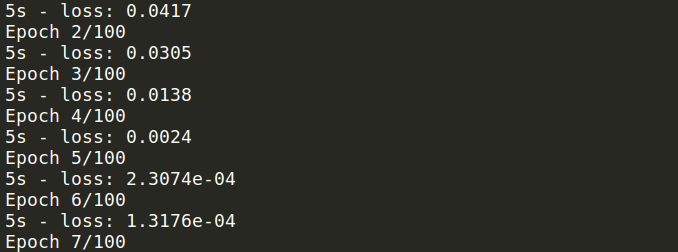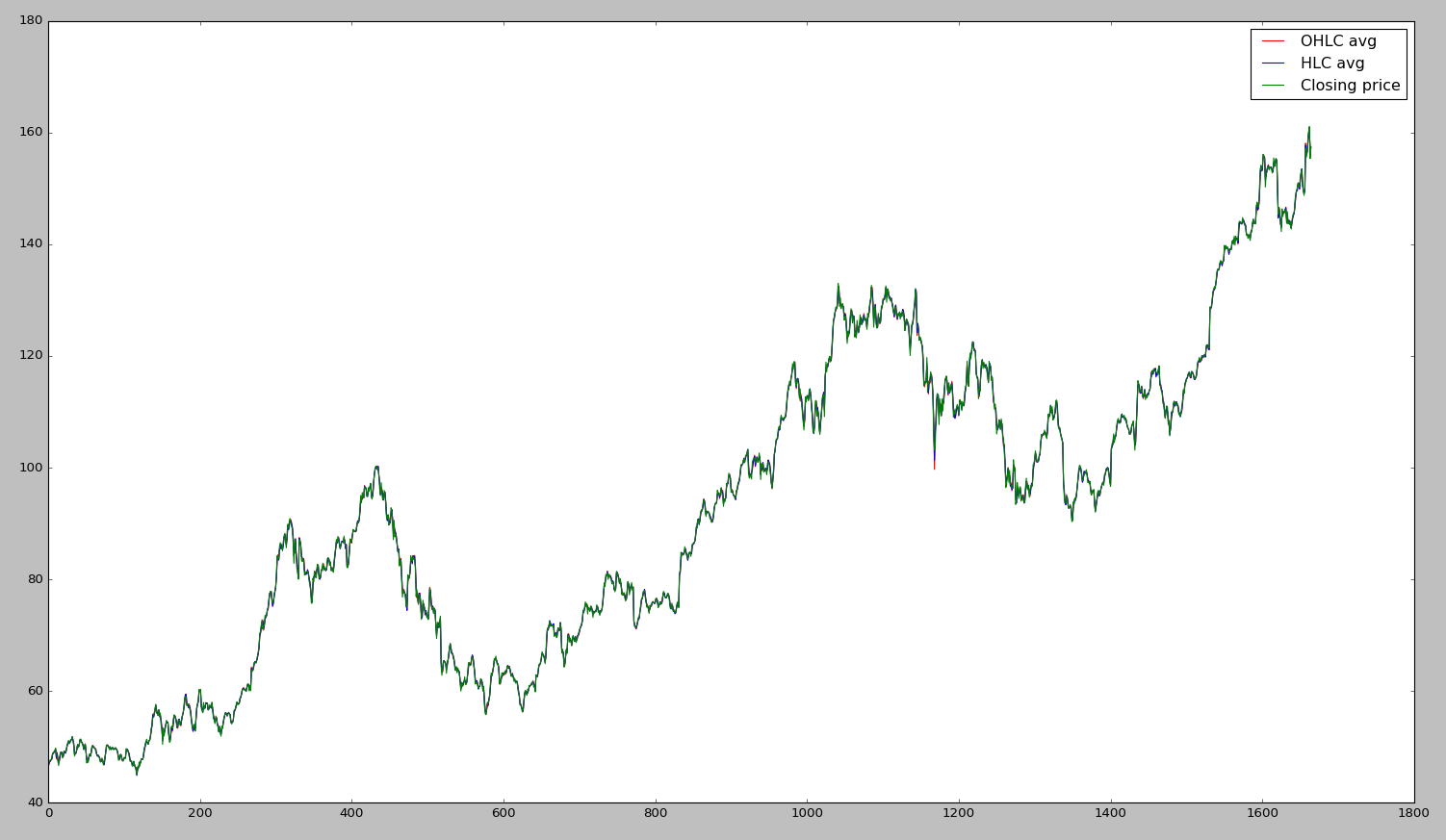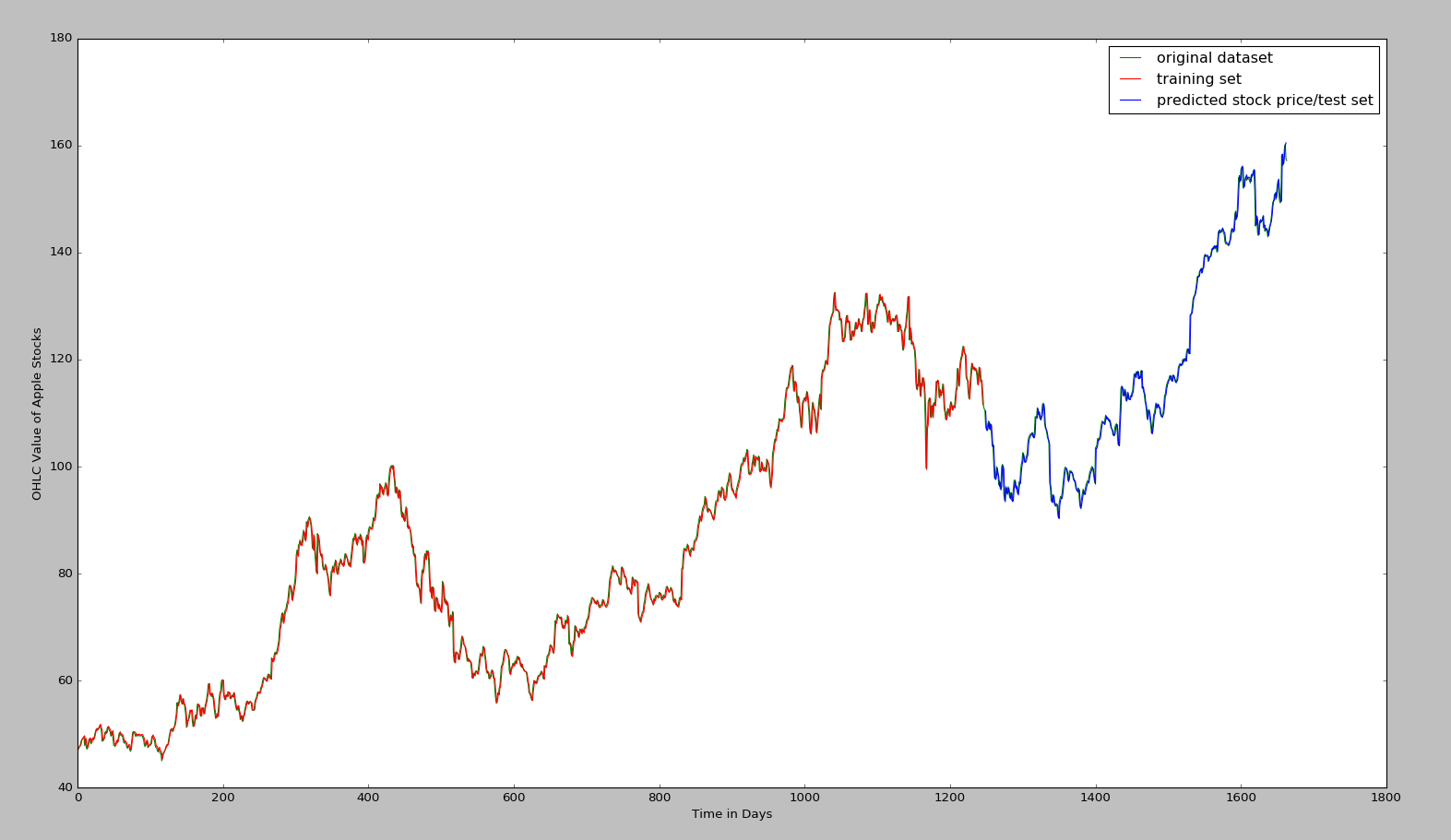# Stock Price Prediction of Apple Inc. Using Recurrent Neural Network

OHLC Average Prediction of Apple Inc. Using LSTM Recurrent Neural Network

# Dataset:

The dataset is taken from yahoo finace's website in CSV format. The dataset consists of Open, High, Low and Closing Prices of Apple Inc. stocks from 3rd january 2011 to 13th August 2017 - total 1664 rows.

# Price Indicator:

Stock traders mainly use three indicators for prediction: OHLC average (average of Open, High, Low and Closing Prices), HLC average (average of High, Low and Closing Prices) and Closing price, In this project, OHLC average has been used.

# Data Pre-processing:

After converting the dataset into OHLC average, it becomes one column data. This has been converted into two column time series data, 1st column consisting stock price of time t, and second column of time t+1. All values have been normalized between 0 and 1.

# Model:

Two sequential LSTM layers have been stacked together and one dense layer is used to build the RNN model using Keras deep learning library. Since this is a regression task, 'linear' activation has been used in final layer.

# Version:

Python 2.7 and latest versions of all libraries including deep learning library Keras and Tensorflow.

# Training:

75% data is used for training. Adagrad (adaptive gradient algorithm) optimizer is used for faster convergence. After training starts it will look like:# Test:

Test accuracy metric is root mean square error (RMSE).

# Results:

The comparison of OHLC, HLC and Closing price:After the training the fitted curve with original stock price:# Observation and Conclusion:

Since difference among OHLC average, HLC average and closing value is not significat, so only OHLC average is used to build the model and prediction. The training and testing RMSE are: 1.24 and 1.37 respectively which is pretty good to predict future values of stock. Stock price of last day of dataset was 158.8745 and using this model and price of next two days are predicted as 160.3230 and 160.9240 - which were 159.2075 and 159.8325 on 14th and 15th August 2017 according to Yahoo Finance. However, future values for any time period can be predicted using this model.

Finally, this work can greatly help the quantitative traders to take decisions.

## GitHub

https://github.com/NourozR/Stock-Price-Prediction-LSTM